GeeksforGeeks App
Open AppBrowser
Continue

# Split a text column into two columns in Pandas DataFrame

Let’s see how to split a text column into two columns in Pandas DataFrame.

Method #1 : Using `Series.str.split()` functions.

Split Name column into two different columns. By default splitting is done on the basis of single space by `str.split()` function.

 `# import Pandas as pd``import` `pandas as pd``  ` `# create a new data frame``df ``=` `pd.DataFrame({``'Name'``: [``'John Larter'``, ``'Robert Junior'``, ``'Jonny Depp'``],``                   ``'Age'``:[``32``, ``34``, ``36``]})``  ` `print``(``"Given Dataframe is :\n"``,df)``  ` `# bydefault splitting is done on the basis of single space.``print``(``"\nSplitting 'Name' column into two different columns :\n"``,``                                  ``df.Name.``str``.split(expand``=``True``))`

Output :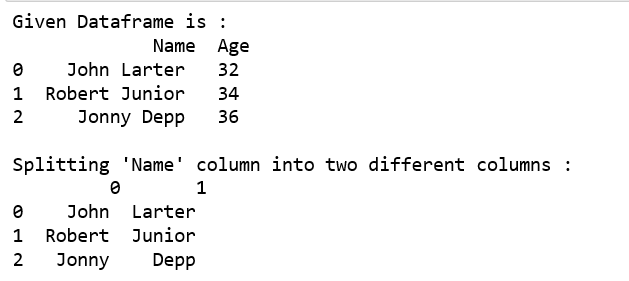Split Name column into “First” and “Last” column respectively and add it to the existing Dataframe .

 `# import Pandas as pd``import` `pandas as pd``  ` `# create a new data frame``df ``=` `pd.DataFrame({``'Name'``: [``'John Larter'``, ``'Robert Junior'``, ``'Jonny Depp'``],``                    ``'Age'``:[``32``, ``34``, ``36``]})``  ` `print``(``"Given Dataframe is :\n"``,df)``  ` `# Adding two new columns to the existing dataframe.``# bydefault splitting is done on the basis of single space.``df[[``'First'``,``'Last'``]] ``=` `df.Name.``str``.split(expand``=``True``)``  ` `print``(``"\n After adding two new columns : \n"``, df)`

Output: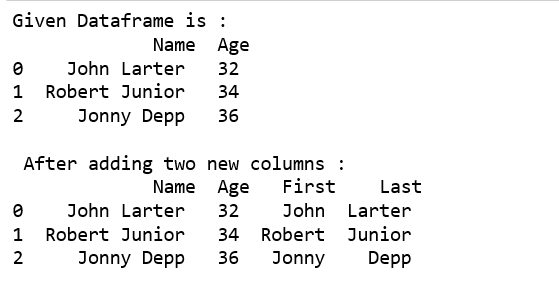Use underscore as delimiter to split the column into two columns.

 `# import Pandas as pd``import` `pandas as pd``  ` `# create a new data frame``df ``=` `pd.DataFrame({``'Name'``: [``'John_Larter'``, ``'Robert_Junior'``, ``'Jonny_Depp'``],``                    ``'Age'``:[``32``, ``34``, ``36``]})``  ` `print``(``"Given Dataframe is :\n"``,df)``  ` `# Adding two new columns to the existing dataframe.``# splitting is done on the basis of underscore.``df[[``'First'``,``'Last'``]] ``=` `df.Name.``str``.split(``"_"``,expand``=``True``)``  ` `print``(``"\n After adding two new columns : \n"``,df)`

Output :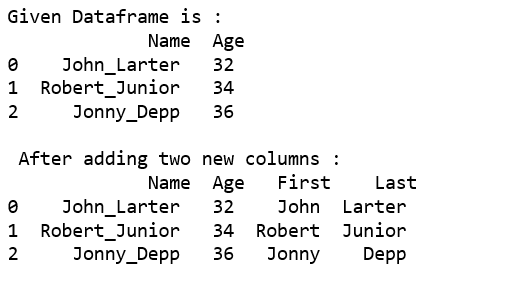Use `str.split()`, `tolist()` function together.

 `# import Pandas as pd``import` `pandas as pd``  ` `# create a new data frame``df ``=` `pd.DataFrame({``'Name'``: [``'John_Larter'``, ``'Robert_Junior'``, ``'Jonny_Depp'``],``                    ``'Age'``:[``32``, ``34``, ``36``]})``  ` `print``(``"Given Dataframe is :\n"``,df)`` ` `print``(``"\nSplitting Name column into two different columns :"``) ``print``(pd.DataFrame(df.Name.``str``.split(``'_'``,``1``).tolist(),``                         ``columns ``=` `[``'first'``,``'Last'``]))`

Output :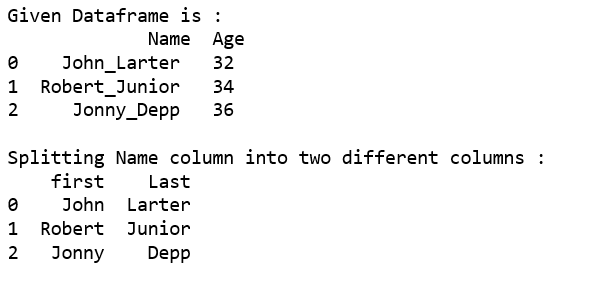Method #2 : Using `apply()` function.

Split Name column into two different columns.

 `# import Pandas as pd``import` `pandas as pd``  ` `# create a new data frame``df ``=` `pd.DataFrame({``'Name'``: [``'John_Larter'``, ``'Robert_Junior'``, ``'Jonny_Depp'``],``                    ``'Age'``:[``32``, ``34``, ``36``]})``  ` `print``(``"Given Dataframe is :\n"``,df)`` ` `print``(``"\nSplitting Name column into two different columns :"``) ``print``(df.Name.``apply``(``lambda` `x: pd.Series(``str``(x).split(``"_"``))))`

Output :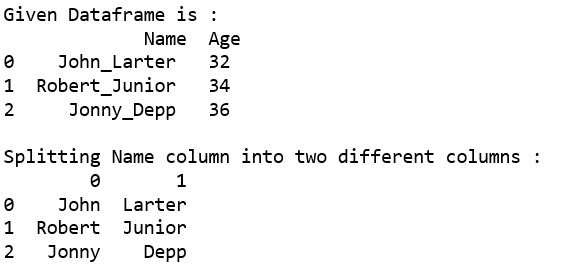Split Name column into two different columns named as “First” and “Last” respectively and then add it to the existing Dataframe.

 `# import Pandas as pd``import` `pandas as pd``  ` `# create a new data frame``df ``=` `pd.DataFrame({``'Name'``: [``'John_Larter'``, ``'Robert_Junior'``, ``'Jonny_Depp'``],``                    ``'Age'``:[``32``, ``34``, ``36``]})``  ` `print``(``"Given Dataframe is :\n"``,df)`` ` `print``(``"\nSplitting Name column into two different columns :"``) `` ` `# splitting 'Name' column into Two columns ``# i.e. 'First' and 'Last'respectively and ``# Adding these columns to the existing dataframe.``df[[``'First'``,``'Last'``]] ``=` `df.Name.``apply``(``   ``lambda` `x: pd.Series(``str``(x).split(``"_"``)))``  ` `print``(df)`

Output :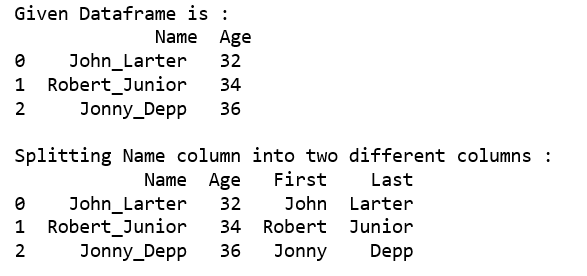My Personal Notes arrow_drop_up